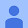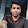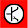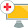AC voltage Part 2: root-mean-square (RMS) value explained in Python

In the second video of the AC power series the basic mathematics behind calculating the root-mean-squared (RMS) value is being explained step-by-step. Furthermore, the process is also shown in form of a Python 3 code run inside a Jupyter notebook. First we describe the general formula for a sinusoidal function, and this is being implemented for 230V AC voltage, having a value of the peak amplitude of about 325V.

For simplicity the code does not contain Numpy arrays, vectorized operations,…

5 thoughts on “AC voltage Part 2: root-mean-square (RMS) value explained in Python”

1.colorado wilderness says:

Actually your works are mesmerizing .

2.Varun Panikar says:

but one request you should do more practical things than theory lessons

3.Elektronik Atölyem says:

Useful video 👍

4.Jim Ballard says:

I like how you went "full Heisenberg" on that equation.

5.Varun Panikar says:

nice way to find out rms value using programming